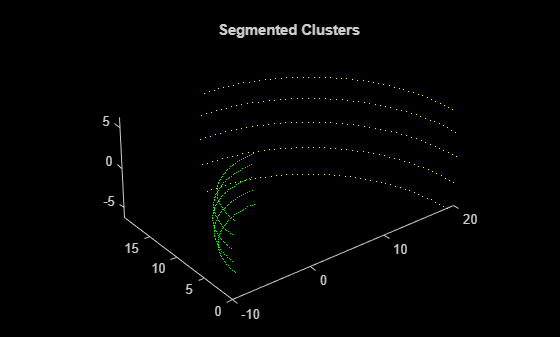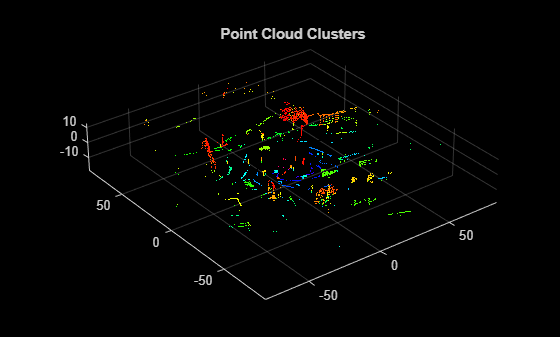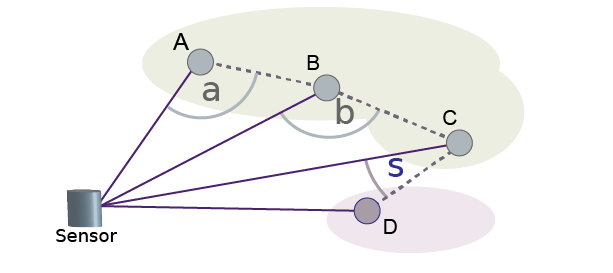# segmentLidarData

Segment organized 3-D range data into clusters

## Syntax

``labels = segmentLidarData(ptCloud,distThreshold)``
``labels = segmentLidarData(ptCloud,distThreshold,angleThreshold)``
``[labels,numClusters] = segmentLidarData(___)``
``[___] = segmentLidarData(___,NumClusterPoints=[1,Inf])``

## Description

example

````labels = segmentLidarData(ptCloud,distThreshold)` segments organized 3-D range data `ptCloud` into clusters. The function assigns an integer cluster label to each point in the point cloud and returns the cluster label of all points in `labels`. The function groups two neighboring points into the same cluster if their Euclidean distance is less than `distThreshold` or if the angle between the sensor and two neighboring points is at least 5 degrees.```

example

````labels = segmentLidarData(ptCloud,distThreshold,angleThreshold)` sets the angle constraint for grouping points into the same cluster to `angleThreshold`.```
````[labels,numClusters] = segmentLidarData(___)` also returns the number of clusters.```
````[___] = segmentLidarData(___,NumClusterPoints=[1,Inf])` also sets the minimum and maximum number of points in each cluster, specified as a 2-element vector or as a scalar value. When you specify `NumClusterPoints` as a scalar, the maximum number of points in the cluster is unrestricted. The function sets the `labels` to `0` when clusters are outside of the specified range.```

## Examples

collapse all

Create organized synthetic lidar data containing two objects.

```ldr = zeros(5,100); ldr(:,1:50) = 10; ldr(:,51:end) = 20; pitch = linspace(-18,18,5); pitch = repmat(pitch',1,100); yaw = linspace(-90,90,100); yaw = repmat(yaw,5,1); ```

Convert to Cartesian coordinates.

```X = ldr .* cosd(pitch) .* sind(yaw); Y = ldr .* cosd(pitch) .* cosd(yaw); Z = ldr .* sind(pitch); pc = pointCloud(cat(3,X,Y,Z)); figure pcshow(pc.Location,'r') title('Unclustered Point Cloud')```Set the threshold.

`distThreshold = 5;`

Segment the lidar data.

`labels = segmentLidarData(pc,distThreshold);`

Plot the results.

```figure hold on title('Segmented Clusters') pc1 = select(pc,find(labels == 1)); pcshow(pc1.Location,'g') pc2 = select(pc,find(labels == 2)); pcshow(pc2.Location,'y')```Set up the PCAP file reader.

`veloReader = velodyneFileReader('lidarData_ConstructionRoad.pcap','HDL32E');`

Wait for 0.3 seconds from the beginning of the file, then read the point cloud from the next frame.

```veloReader.CurrentTime = veloReader.StartTime + seconds(0.3); ptCloud = readFrame(veloReader);```

Segment and remove the ground plane.

```groundPtsIdx = segmentGroundFromLidarData(ptCloud); ptCloudWithoutGround = select(ptCloud,~groundPtsIdx,'OutputSize','full');```

Cluster the remaining points. Distance is in meters.

```distThreshold = 0.5; [labels,numClusters] = segmentLidarData(ptCloudWithoutGround,distThreshold);```

```numClusters = numClusters+1; labels(groundPtsIdx) = numClusters;```

Plot the labeled results. Display the ground plane in black.

```labelColorIndex = labels+1; pcshow(ptCloud.Location,labelColorIndex) colormap([hsv(numClusters);[0 0 0]]) title('Point Cloud Clusters')```## Input Arguments

collapse all

Point cloud, specified as a `pointCloud` object. `ptCloud` is an organized point cloud that stores point coordinates in an M-by-N-by-3 matrix. The points must be organized by pitch and yaw angles in a sequential scanning order, which is typically obtained from laser range finders and the `velodyneFileReader`.

Distance threshold in world units, specified as a nonnegative scalar. Adjacent points are grouped into the same cluster if the distance between them is less than the distance threshold. To reduce the number of output clusters, increase the value of `distThreshold`.

Data Types: `single` | `double`

Angle threshold, in degrees, specified as a scalar in the range [0, 180]. To reduce the number of output clusters, decrease the value of `angleThreshold`. The function groups adjacent points into the same cluster if the angle formed by the sensor and the points is greater than the angle threshold. For example, in the figure, the function groups points A and B into the same cluster if the angle formed by the sensor, point A, and point B, is greater than `angleThreshold`. For more details, see Algorithms.Data Types: `single` | `double`

## Output Arguments

collapse all

Cluster labels of all points in the point cloud `ptCloud`, returned as an M-by-N matrix of integers. Each valid point in `ptCloud` belongs to a cluster. All points in a cluster are assigned the same integer cluster label, ranging from `1` to `numClusters`. Invalid points, such as points with `Inf` or `NaN` coordinates, are assigned the label `0`.

Number of clusters, returned as a positive integer. The number of clusters does not include the cluster corresponding to invalid points and excludes the label value, `0`, which is reserved for invalid points.

## Algorithms

The `segmentLidarData` function uses distance and angle thresholds to cluster neighboring points. The function groups two neighboring points into the same cluster if their Euclidean distance is less than the input `distThreshold` or if the angle between the sensor and neighboring points is greater than or equal to the input `angleThreshold`. If you do not specify `angleThreshold`, the function sets this angle to `5` degrees.

For example, suppose `angleThreshold` is set to `90`. Because angles α and β in the figure are both greater than the specified threshold of 90 degrees, the function groups points A, B, and C into the same cluster. Because angle σ is less than the 90-degree threshold, the function groups point D into a separate cluster. Each angle the function uses for clustering is formed by the line from a point to the sensor and the line from that same point to the neighboring pointBogoslavskyi, I. “Efficient Online Segmentation for Sparse 3D Laser Scans.” Journal of Photogrammetry, Remote Sensing and Geoinformation Science. Vol. 85, Issue 1, 2017, pp. 41–52.